# Intermediate Maths Solutions for Product of Vectors Exercise 5(c)

Inter Maths 1A text book Product of Vectors Exercise 5(c) solutions are given.

Study the text book lesson Product of Vectors very well.

Observe the example problems and solutions in the text book. Try them well.

Observe the given below solutions and try them in your own method.

You can also see the solutions

SSC Maths text book Solutions class 10

Inter maths 1A textbook solutions

Inter Maths 1B textbook solutions

Inter Maths 1IA text book solutions

Inter Maths IIB text book solutions

Product of Vectors

Exercise 5(a)

Exercise 5(b)

Exetcise 5(c)

M

# Inter Maths Solutions for Product of Vectors Exercise 5(c)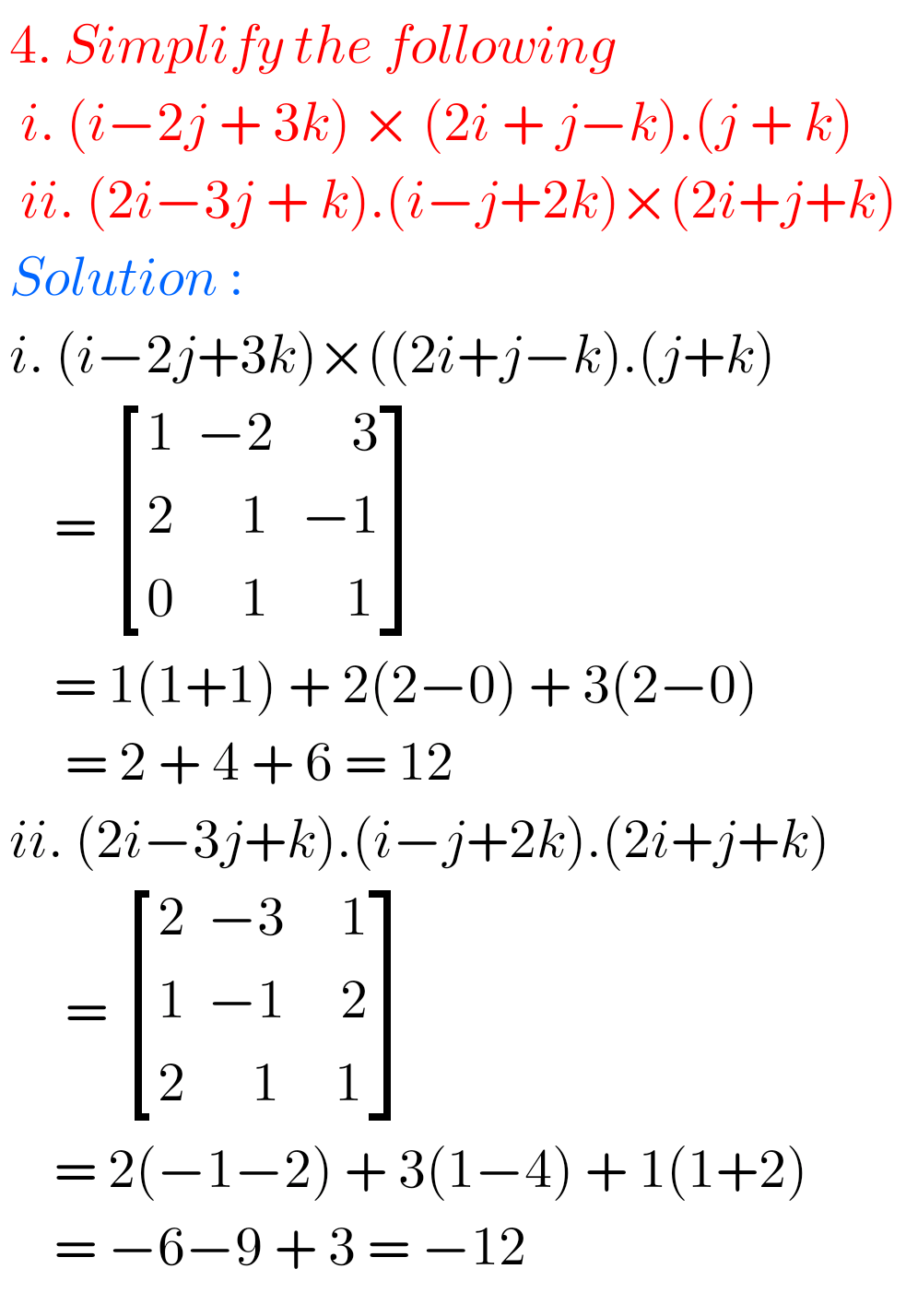##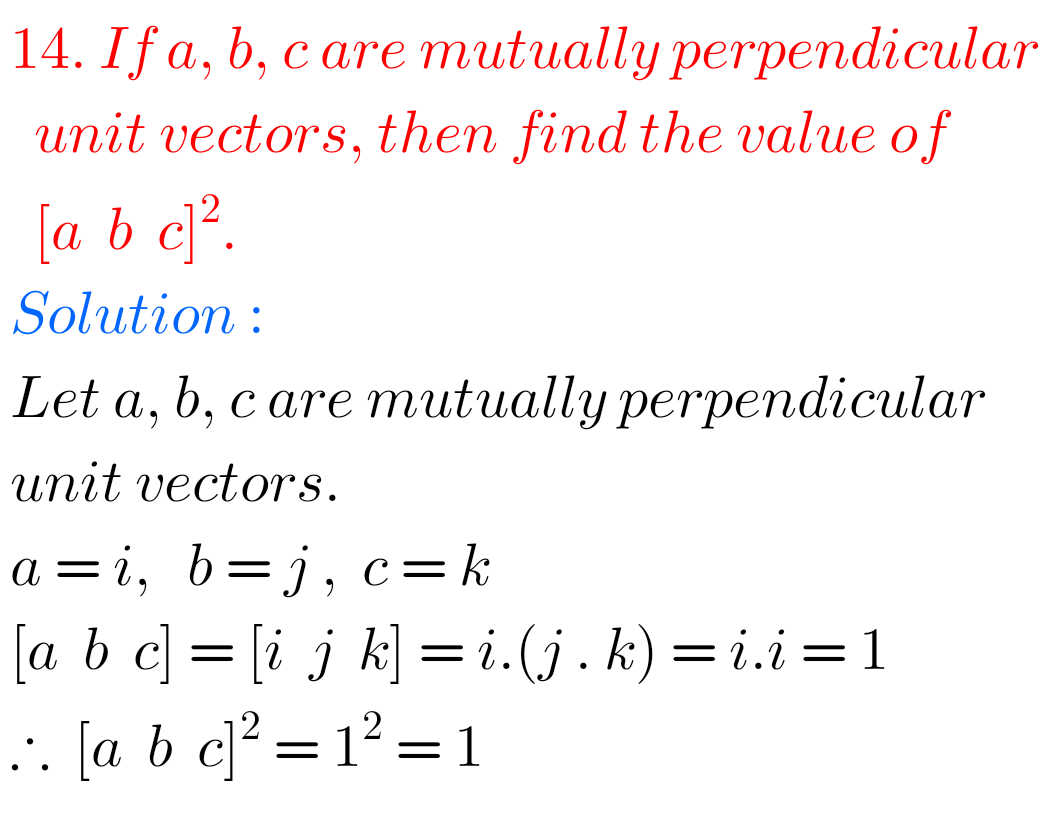## Product of Vectors Solutions Exercise 5(c)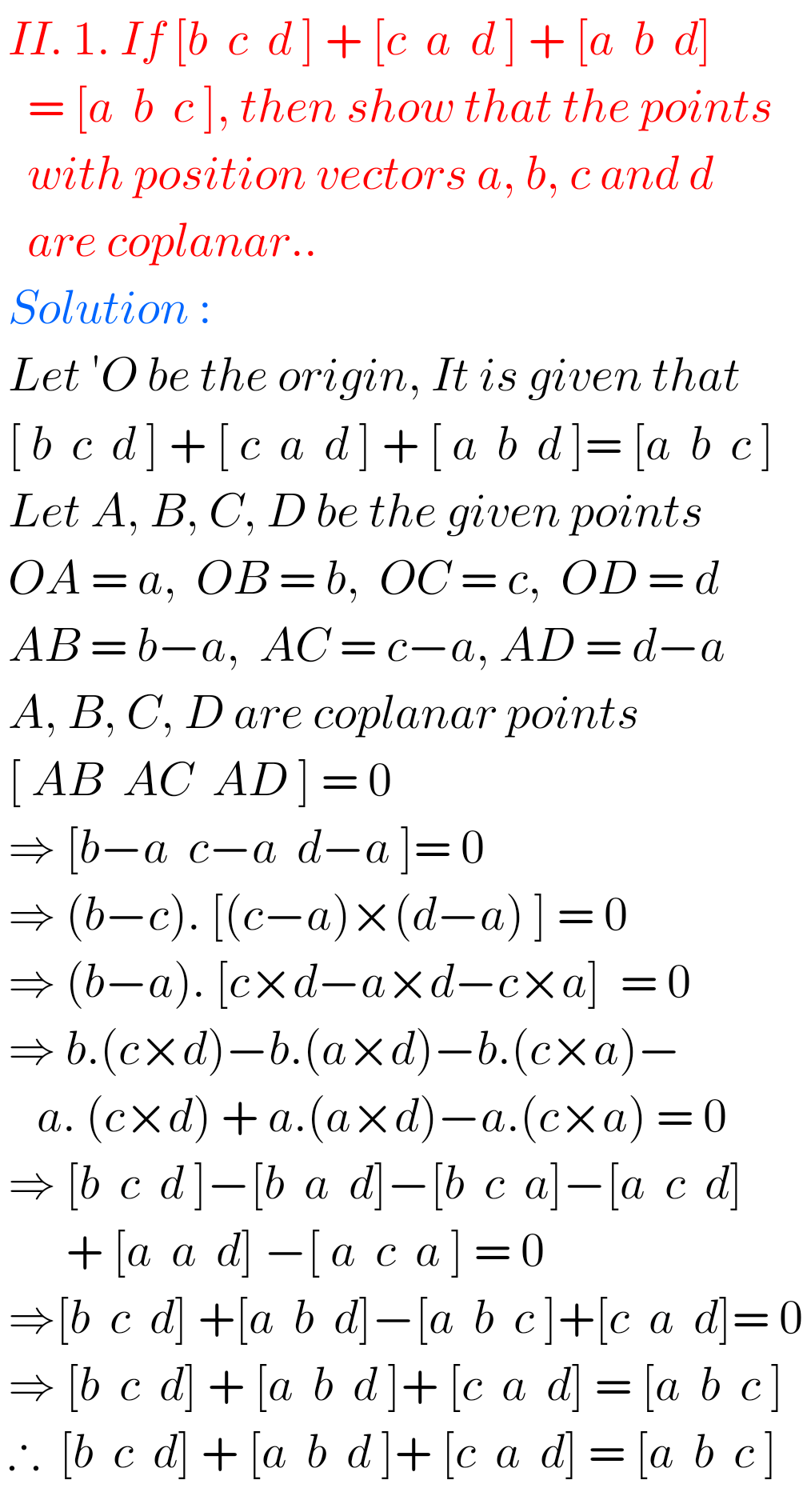##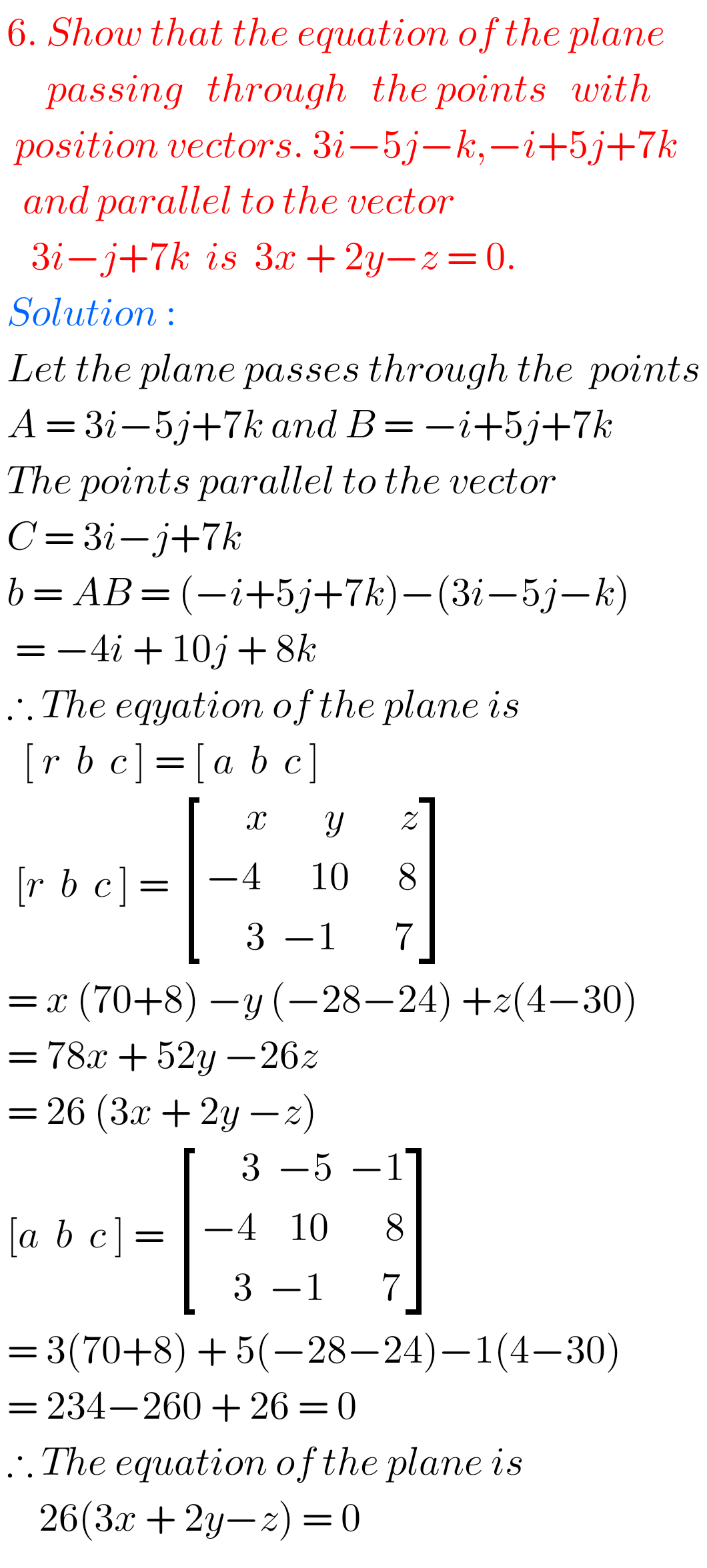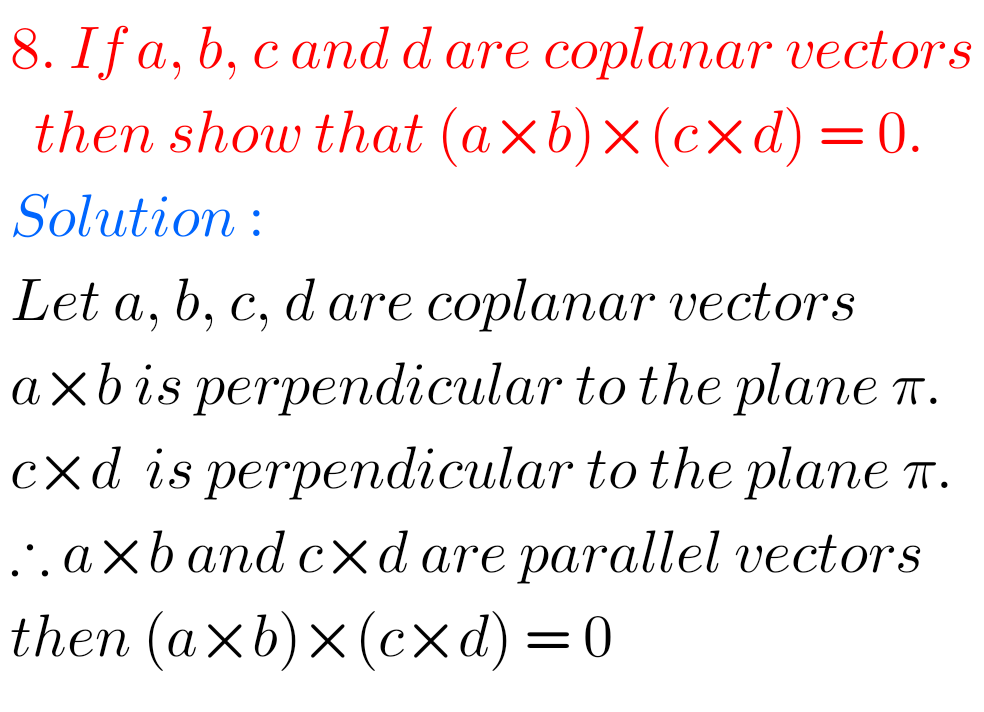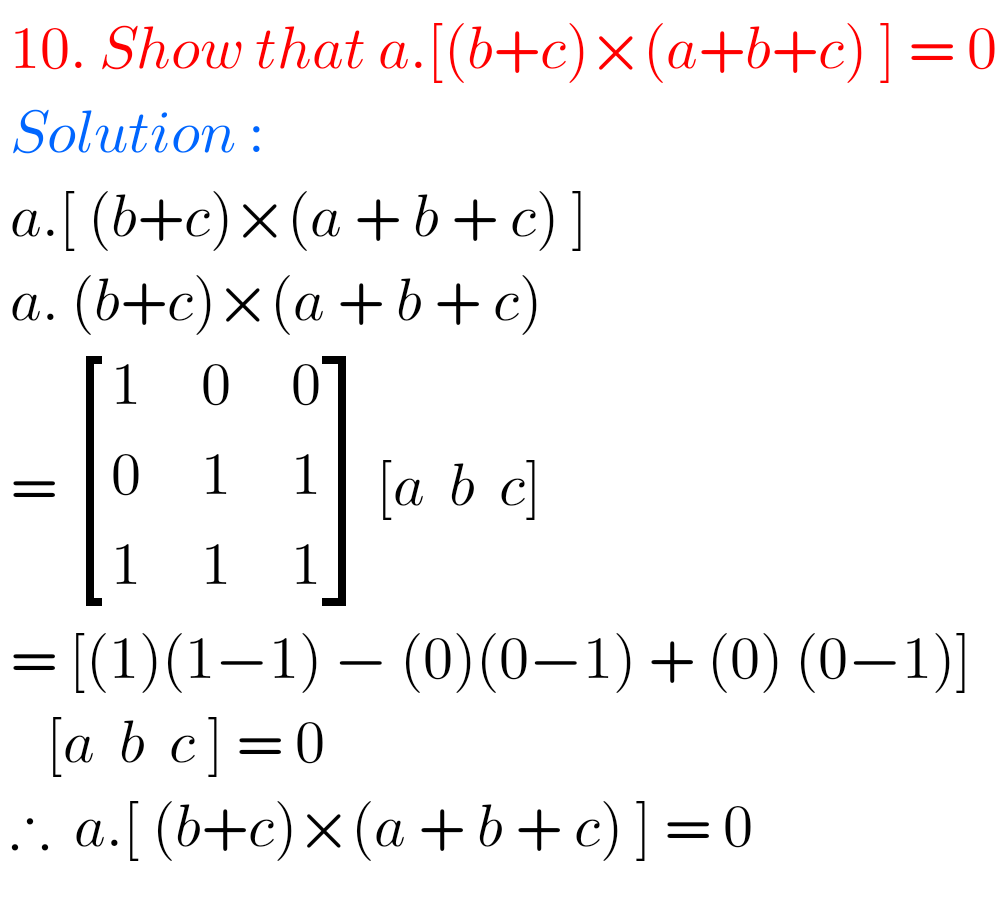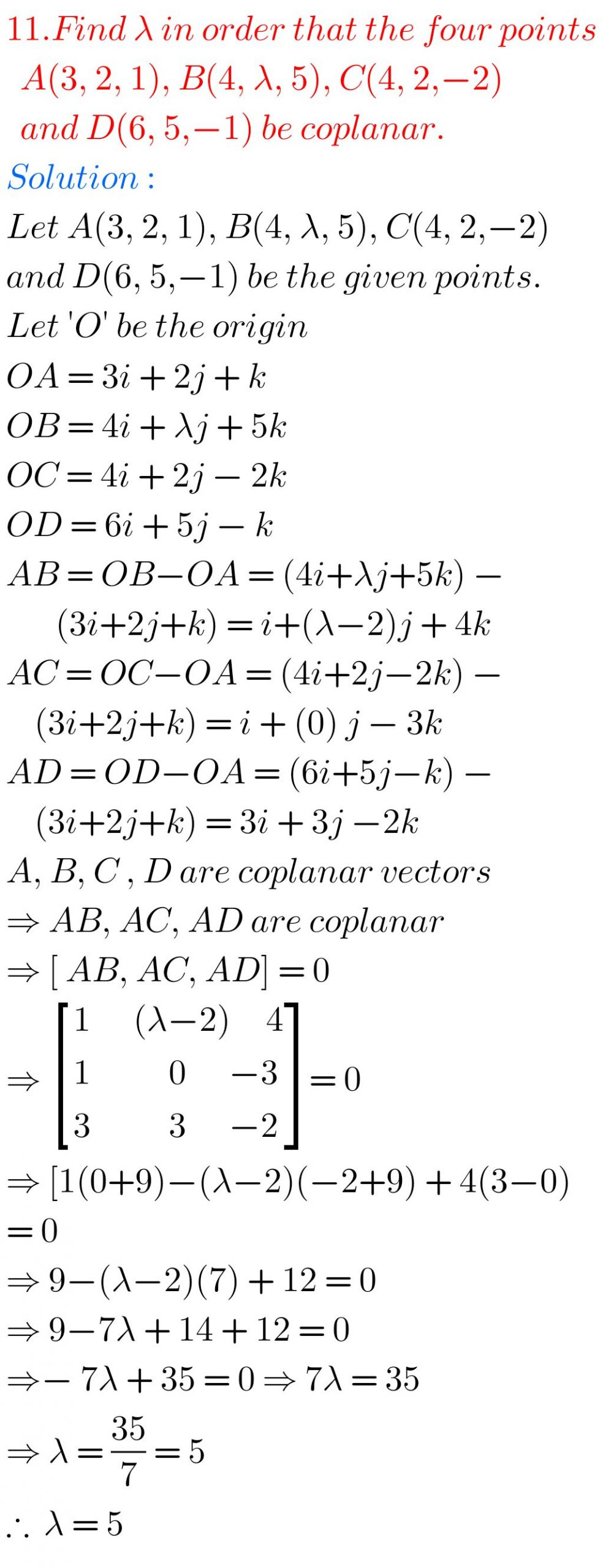## Maths Solutions for Product of Vectors Exercise 5(c)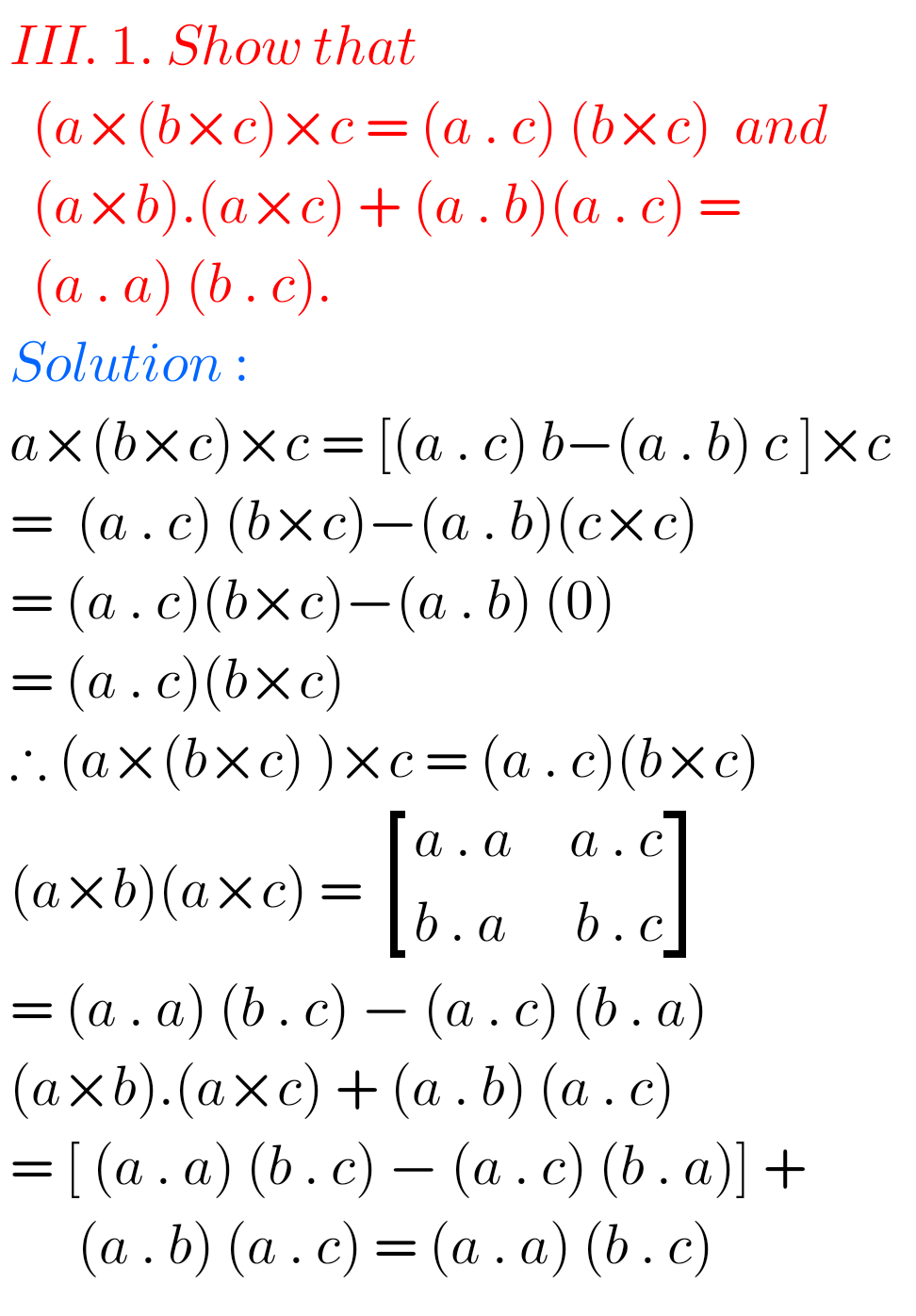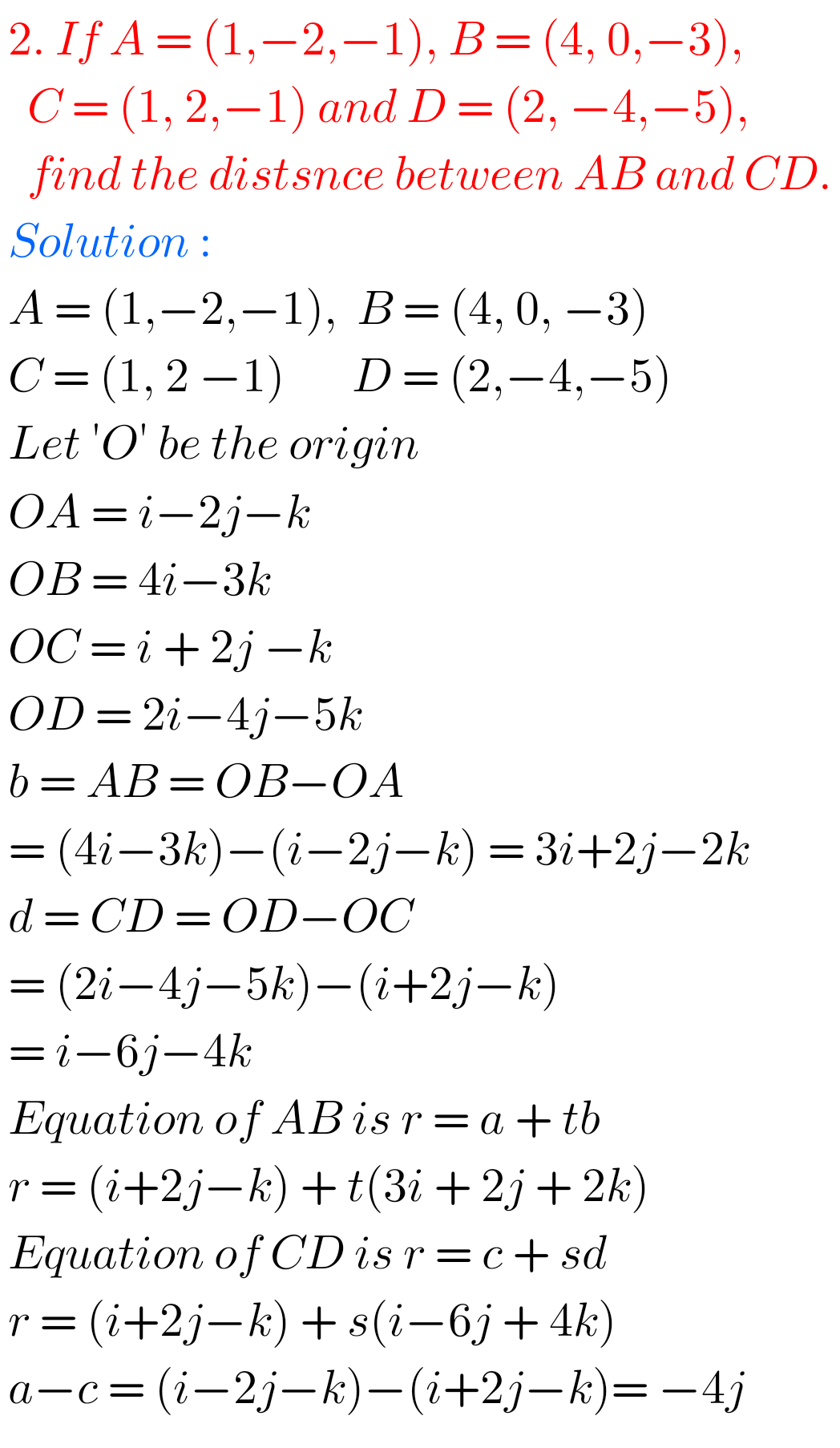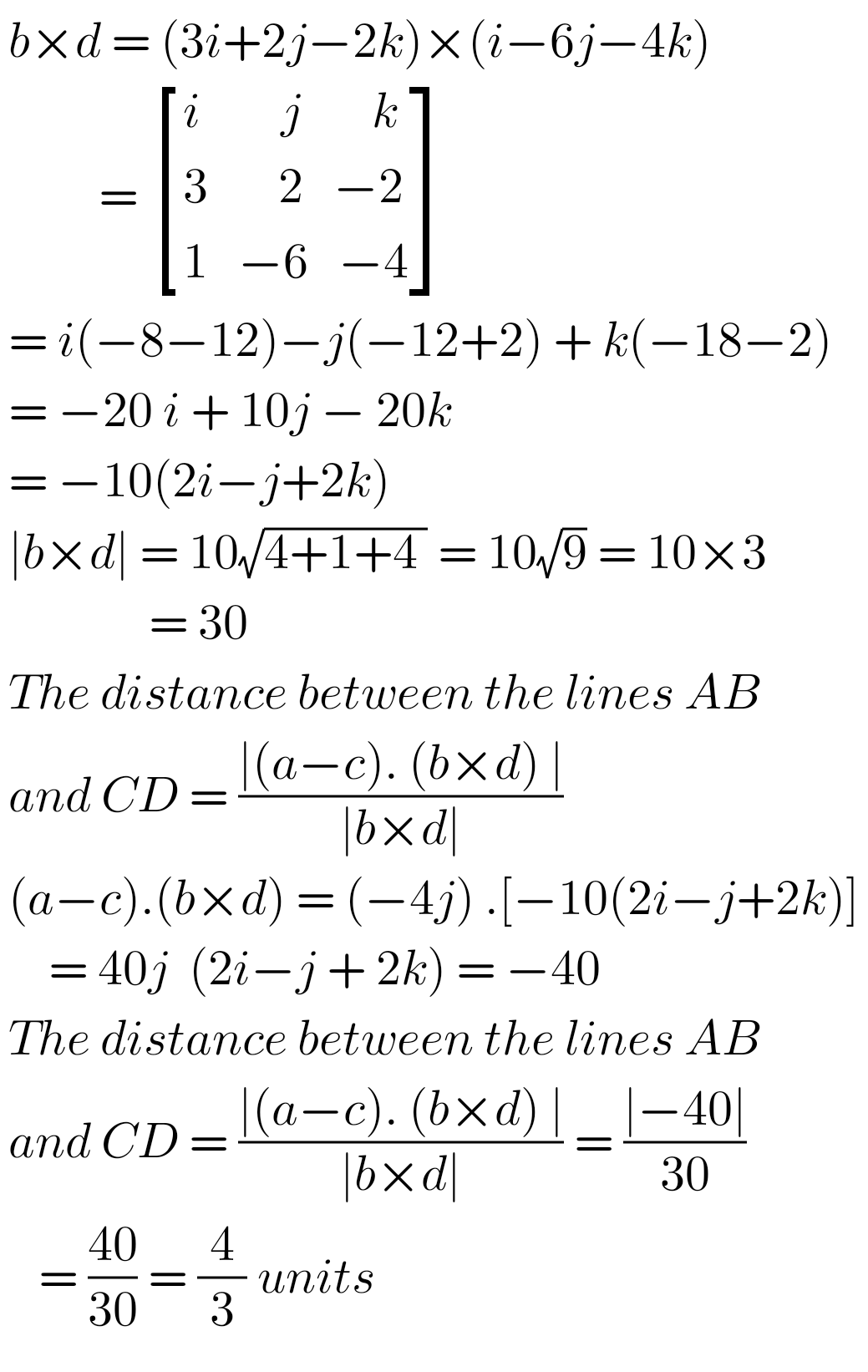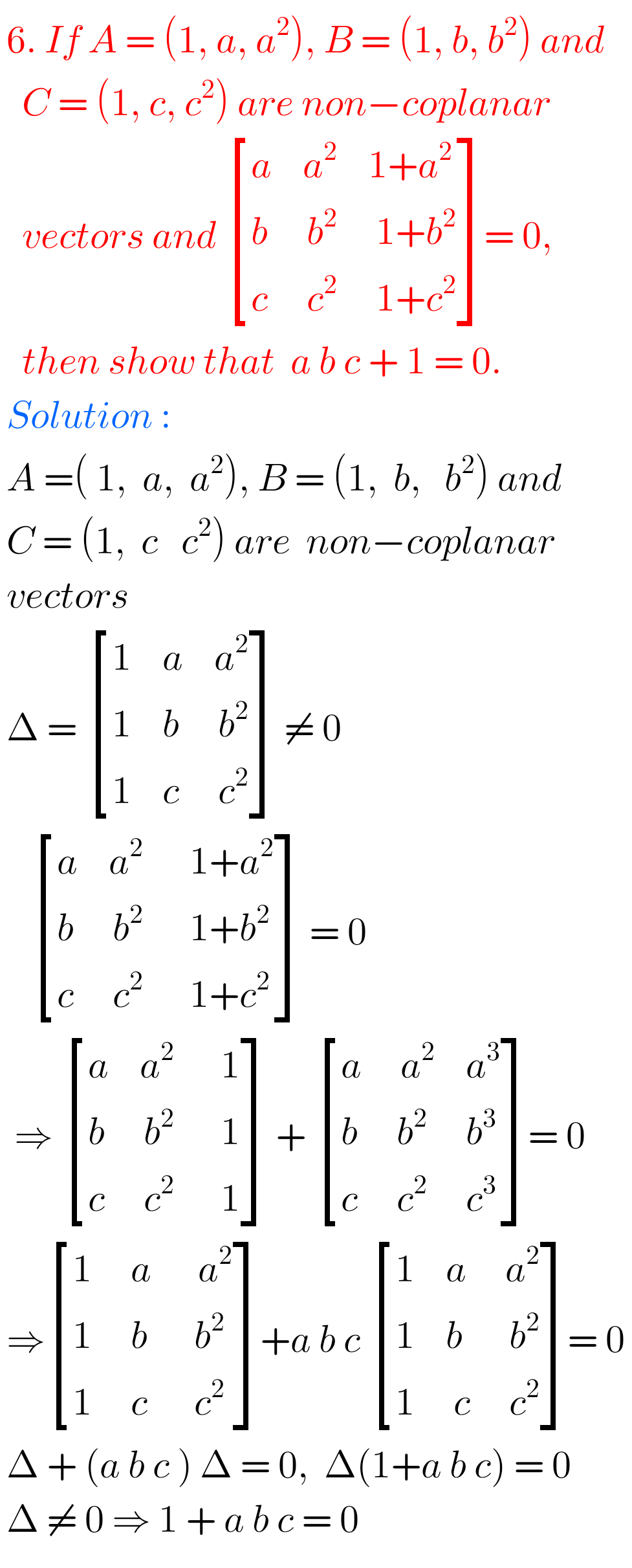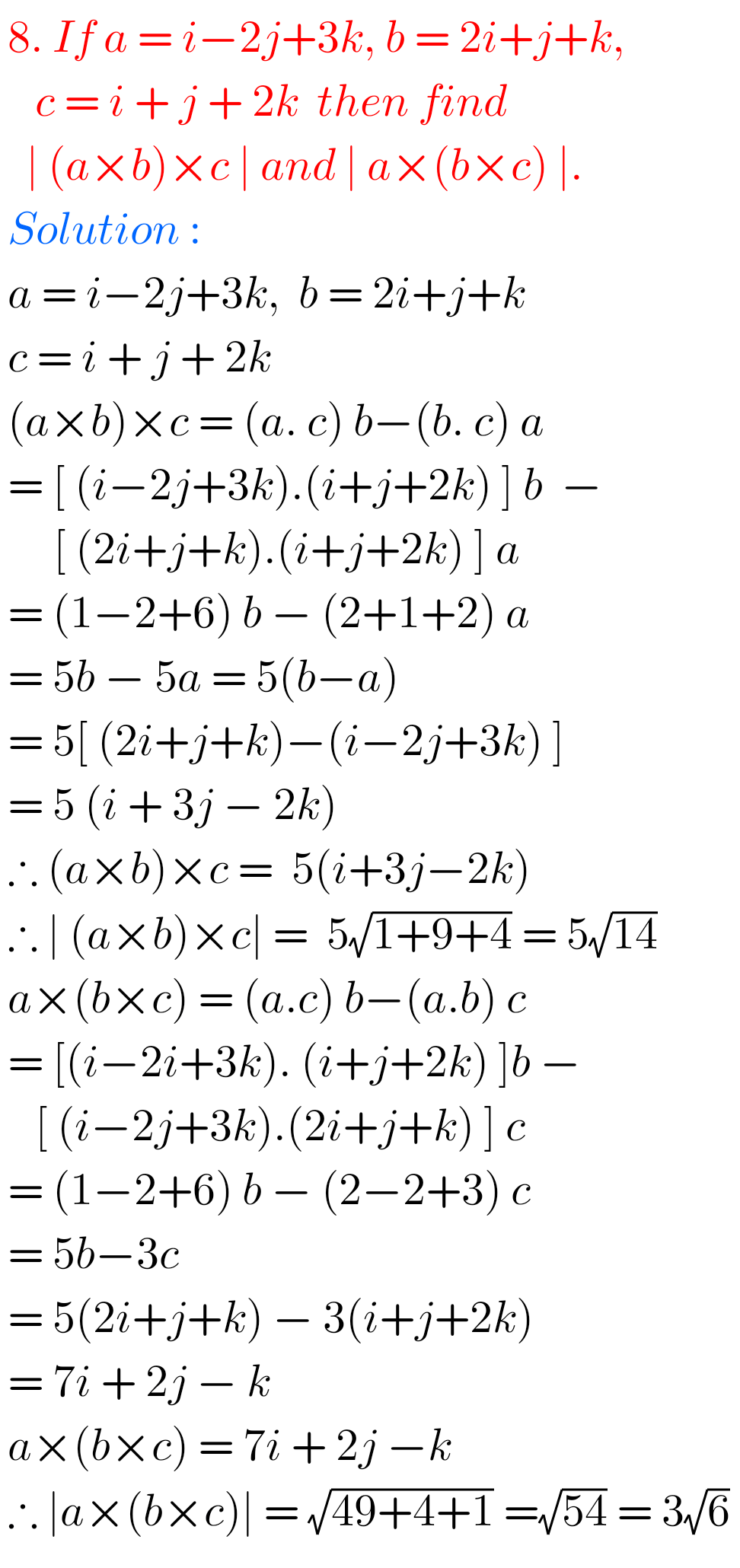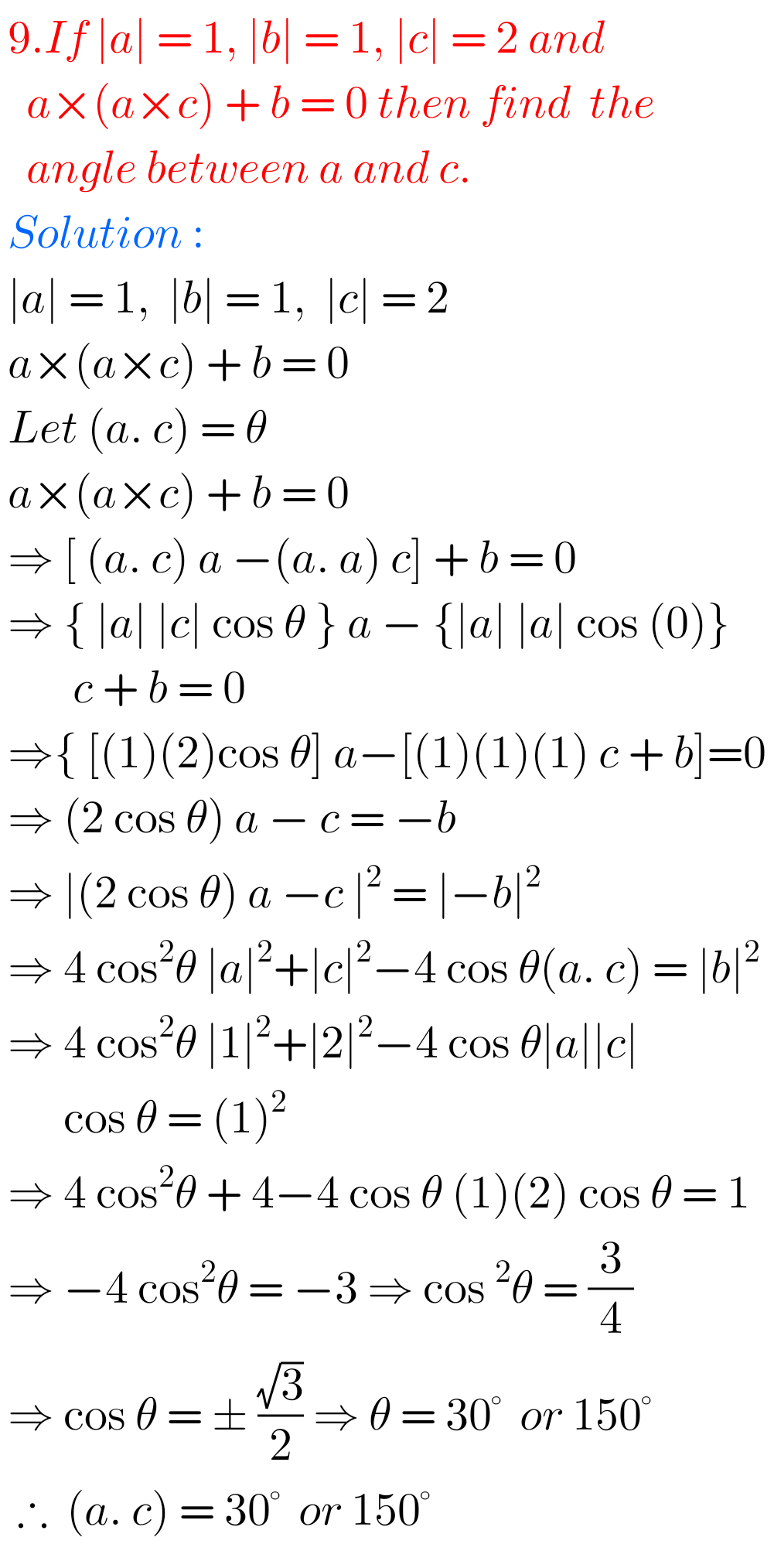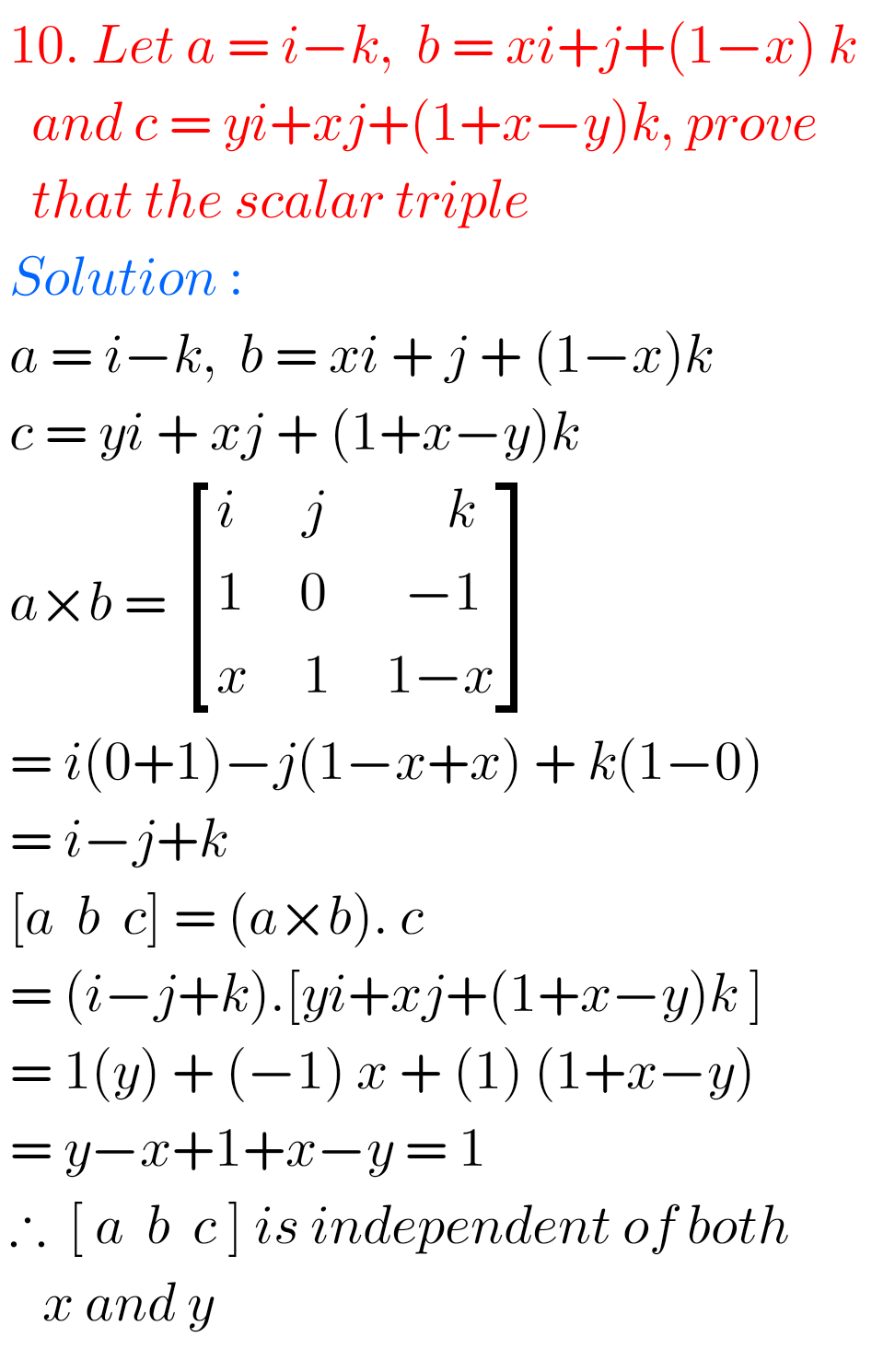Note : Observe the solutions and try them in your own method.

### 3 thoughts on “Intermediate Maths Solutions for Product of Vectors Exercise 5(c)”

1.Nice

2.G.keerthana GORANTLA

Thanks for this this is very helpful to understand the concept 😃😃☺️

3.Thanks for this this website is very helpful to understand the concept 😃😃☺️